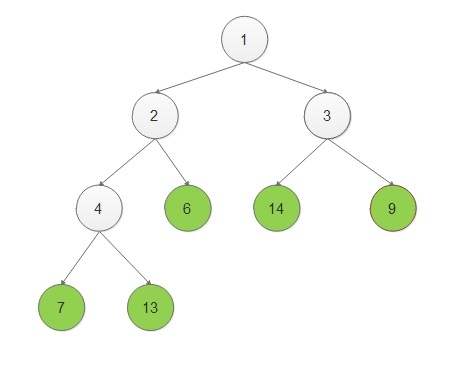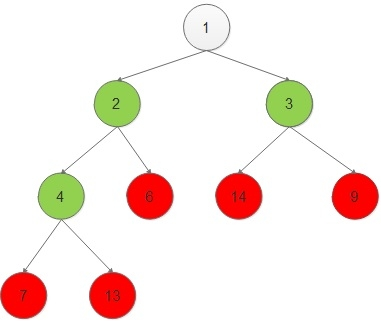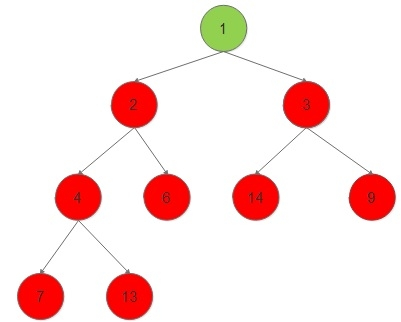# Print the nodes of binary tree as they become the leaf node in C++ Programming.

Given a binary tree, we have to print its leaf nodes and then we have to remove those leaf nodes and then repeat till there are no nodes left in the tree.

## ExampleSo the output of the problem should be −

6 7 9 13 14
3 4
2
1

## Approach

We have adopted an approach where we are applying DFS.

For applying a temporary value zero is assigned to every value and then assign all the nodes with the value maximum(value of both child)+1.

## Algorithm

START
STEP 1-> DEFINE A struct Node
WITH DATA MEMBERS data, order, *left, *right
STEP 2-> DEFINE A struct Node* newNode(int data, int order)
WITH struct Node* node = new Node, node->data = data, node->order = order,
node->left = NULL, node->right = NULL, return (node)
FUNCTION void postod(struct Node* node, vector<pair<int, int> >& v)
STEP 1-> IF node == NULL THEN,
RETURN
STEP 2-> CALL FUNCTION postod(node->left, v)
STEP 3-> CALL FUNCTION postod(node->right, v)
STEP 4-> IF node->right == NULL && node->left == NULL THEN,
SET node->order AS 1
v.push_back(make_pair(node->order, node->data))
ELSE
node->order = max((node->left)->order, (node->right)->order) + 1
v.push_back(make_pair(node->order, node->data))
END IF
FUNCTION void printLeafNodes(int n, vector<pair<int, int> >& v)
STEP 1-> sort(v.begin(), v.end())
STEP 2-> LOOP FOR i = 0 AND i < n AND i++
IF v[i].first == v[i + 1].first THEN,
PRINT v[i].second
ELSE
PRINT v[i].second
END IF
END FOR
IN main()
STEP 1-> CREATE A ROOT NODE LIKE struct Node* root = newNode(8, 0)
STEP 2-> DECLARE AND SET n = 9
STEP 3-> CALL postod(root, v);
STEP 4-> CALL printLeafNodes(n, v);

## Example

Live Demo

#include <bits/stdc++.h>
using namespace std;
struct Node {
int data;
int order;
struct Node* left;
struct Node* right;
};
struct Node* newNode(int data, int order){
struct Node* node = new Node;
node->data = data;
node->order = order;
node->left = NULL;
node->right = NULL;
return (node);
}
void postod(struct Node* node, vector<pair<int, int> >& v){
if (node == NULL)
return;
/* first recur on left child */
postod(node->left, v);
/* now recur on right child */
postod(node->right, v);
// If current node is leaf node, it's order will be 1
if (node->right == NULL && node->left == NULL) {
node->order = 1;
// make pair of assigned value and tree value
v.push_back(make_pair(node->order, node->data));
} else {
node->order = max((node->left)->order, (node->right)->order) + 1;
v.push_back(make_pair(node->order, node->data));
}
}
void printLeafNodes(int n, vector<pair<int, int> >& v){
sort(v.begin(), v.end());
for (int i = 0; i < n; i++) {
if (v[i].first == v[i + 1].first)
cout << v[i].second << " ";
else
cout << v[i].second << "\n";
}
}
int main(){
struct Node* root = newNode(1, 0);
root->left = newNode(2, 0);
root->right = newNode(3, 0);
root->left->left = newNode(4, 0);
root->left->right = newNode(6, 0);
root->right->left = newNode(14, 0);
root->right->right = newNode(9, 0);
root->left->left->left = newNode(7, 0);
root->left->left->right = newNode(13, 0);
int n = 9;
vector<pair<int, int> > v;
postod(root, v);
printLeafNodes(n, v);
return 0;
}

## Output

This Program will Print output −

6 7 9 13 14
3 4
2
1